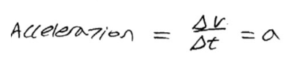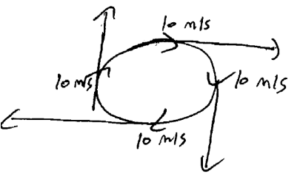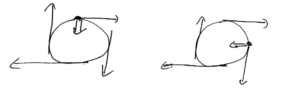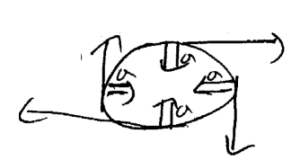Blog / Acceleration

### Acceleration

Written by Chau Pham on Feb 26, 2020

Acceleration is a vector representing the change in velocity with respect to change in time.We can further explore this concept through the example of uniform circular motion.When the object is at 12-o’clock and going to the right, there is a downward push to bring it to the 3 o’clock position. When the object is at 3-o’clock and going to the right, there is a push to the left to bring it to the 6 o’clock position.According to Newton’s Second Law: F = ma, so if there is a force on the object, there must be an acceleration. The velocity is changing either its magnitude or direction or both. In this case where the object is travelling with uniform circular motion, the velocity has constant magnitude with changing direction. As a result, the acceleration’s direction changes but the magnitude remains constant. The acceleration of uniform circular motion always points towards the center.### KEY TAKEAWAYS

Key Points:

• Acceleration is change is velocity over a period of time.
• The acceleration of uniform circular motion always points towards the center.

Key Terms:

• Uniform circular motion: movement of an object along the circumference of a circle with constant speed and changing direction.

Billing Information